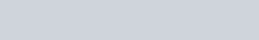Two tiny spheres carrying charges 1.5 μC and 2.5 μC are located 30 cm apart.
Question:

Two tiny spheres carrying charges 1.5 μC and 2.5 μC are located 30 cm apart. Find the potential and electric field:

(a) at the mid-point of the line joining the two charges, and

(b) at a point 10 cm from this midpoint in a plane normal to the line and passing through the mid-point.

Solution:

Two charges placed at points A and B are represented in the given figure. O is the mid-point of the line joining the two charges.Magnitude of charge located at A, q1 = 1.5 μC

Magnitude of charge located at B, q2 = 2.5 μC

Distance between the two charges, d = 30 cm = 0.3 m

(a) Let V1 and E1 are the electric potential and electric field respectively at O.

V1 = Potential due to charge at A + Potential due to charge at B

$V_{1}=\frac{q_{1}}{4 \pi \epsilon_{0}\left(\frac{d}{2}\right)}+\frac{q_{2}}{4 \pi \epsilon_{0}\left(\frac{d}{2}\right)}=\frac{1}{4 \pi \epsilon_{0}\left(\frac{d}{2}\right)}\left(q_{1}+q_{2}\right)$

Where,

0 = Permittivity of free space

$\frac{1}{4 \pi \in_{0}}=9 \times 10^{9} \mathrm{NC}^{2} \mathrm{~m}^{-2}$

$\therefore V_{1}=\frac{9 \times 10^{9} \times 10^{-6}}{\left(\frac{0.30}{2}\right)}(2.5+1.5)=2.4 \times 10^{5} \mathrm{~V}$

$E_{1}=$ Electric field due to $q_{2}$ – Electric field due to $q_{1}$

$=\frac{q_{2}}{4 \pi \in_{0}\left(\frac{d}{2}\right)^{2}}-\frac{q_{1}}{4 \pi \in_{0}\left(\frac{d}{2}\right)^{2}}$

$=\frac{9 \times 10^{9}}{\left(\frac{0.30}{2}\right)^{2}} \times 10^{6} \times(2.5-1.5)$

$=4 \times 10^{5} \mathrm{~V} \mathrm{~m}^{-1}$

Therefore, the potential at mid-point is $2.4 \times 10^{5} \mathrm{~V}$ and the electric field at mid-point is $4 \times 10^{5} \mathrm{~V} \mathrm{~m}^{-1}$. The field is directed from the larger charge to the smaller charge.

(b) Consider a point Z such that normal distanceOZ = 10 cm = 0.1 m, as shown in the following figure.V2 and Eare the electric potential and electric field respectively at Z.

It can be observed from the figure that distance,

$\mathrm{BZ}=\mathrm{AZ}=\sqrt{(0.1)^{2}+(0.15)^{2}}=0.18 \mathrm{~m}$

$V_{2}=$ Electric potential due to $A+$ Electric Potential due to $B$

$=\frac{q_{1}}{4 \pi \in_{0}(\mathrm{AZ})}+\frac{q_{1}}{4 \pi \in_{0}(\mathrm{BZ})}$

$=\frac{9 \times 10^{9} \times 10^{-6}}{0.18}(1.5+2.5)$

$=2 \times 10^{5} \mathrm{~V}$

Electric field due to $q$ at $Z$,b

$E_{\mathrm{A}}=\frac{q_{1}}{4 \pi \in_{0}(\mathrm{AZ})^{2}}$

$=\frac{9 \times 10^{9} \times 1.5 \times 10^{-6}}{(0.18)^{2}}$

$=0.416 \times 10^{6} \mathrm{~V} / \mathrm{m}$

Electric field due to q2 at Z,

$E_{\mathrm{B}}=\frac{q_{2}}{4 \pi \epsilon_{0}(\mathrm{BZ})^{2}}$

$=\frac{9 \times 10^{9} \times 2.5 \times 10^{-6}}{(0.18)^{2}}$

$=0.69 \times 10^{6} \mathrm{Vm}^{-1}$

The resultant field intensity at Z,

$E=\sqrt{E_{\mathrm{A}}^{2}+E_{\mathrm{B}}^{2}+2 E_{\mathrm{A}} E_{\mathrm{B}} \cos 2 \theta}$

Where, 2θis the angle, ∠AZ B

From the figure, we obtain

$\cos \theta=\frac{0.10}{0.18}=\frac{5}{9}=0.5556$

$\theta=\cos ^{-10} 0.5556=56.25$

$\therefore 2 \theta=112.5^{\circ}$

$\cos 2 \theta=-0.38$

$E=\sqrt{\left(0.416 \times 10^{6}\right)^{2} \times\left(0.69 \times 10^{6}\right)^{2}+2 \times 0.416 \times 0.69 \times 10^{12} \times(-0.38)}$

$=6.6 \times 10^{5} \mathrm{Vm}^{-1}$

Therefore, the potential at a point $10 \mathrm{~cm}$ (perpendicular to the mid-point) is $2.0 \times 10^{5} \mathrm{~V}$ and electric field is $6.6 \times 10^{5} \mathrm{~V} \mathrm{~m}^{-1}$.